# Civil Engineering - UPSC Civil Service Exam Questions

11.

Given that for a channel section, the width of flange = b, the depth of the web between centres of flanges = h, the thickness of flange = t(, the moment of interia of the channel about the axis of bending = I, the distance 'e' of the shear centre outside the channel section from the mid-thickness of the web is:

 A.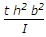B.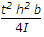C.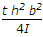D.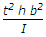Explanation:

No answer description available for this question. Let us discuss.

12.

The effective flange area in tension of a plate girder is equal to (where Af is the area of each flange and Aw, is the web area).

 A. Af B.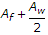C.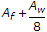D.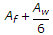Explanation:

No answer description available for this question. Let us discuss.

13.

For complete hydration of cement the W/C ratio needed is

 A. less than 0.25 B. more than 0.25 but less than 0.35 C. more than 0.35 but less than 0.45 D. more than 0.45 but less than 0.60

Explanation:

No answer description available for this question. Let us discuss.

14.

A hollow circular column at internal diameter 'd' and external diameter '1.5 d' is subjected to compressive load. The maximum distance of the point of application of load from the centre for no tension is

 A. d/8 B. 13d/48 C. d/4 D. 13d/96

Explanation:

No answer description available for this question. Let us discuss.

15.

Consider the following statements :
1. Alum coagulation decreases the alkalinity of water.
2. Alum coagulation increases permanent hardness of water.
3. Alum coagulation decreases pH of water.
4. Alum coagulation produces aluminium hydroxide flocks in the flocculation process.
Which of the statements given above are correct ?

 A. 1, 2, 3 and 4 B. 1, 3 and 4 only C. 1 and 2 only D. 2, 3 and 4 only